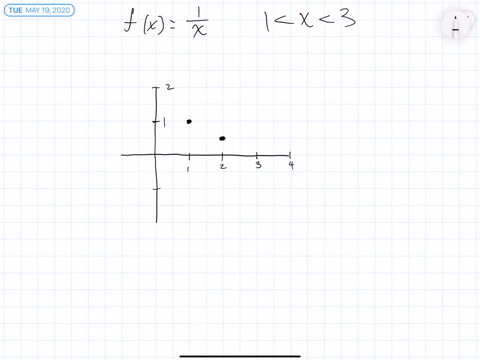Sign up for our free STEM online summer camps starting June 1st!View Summer Courses### $15-22=$ Sketch the graph of $f$ by hand and use …

01:16
ZW
Stetson University

Need more help? Fill out this quick form to get professional live tutoring.

Get live tutoring
Problem 19

$15-22=$ Sketch the graph of $f$ by hand and use your sketch to
find the absolute and local maximum and minimum values of $f .$
(Use the graphs and transformations of Sections $1.2 . )$
$$f(x)=\ln x, \quad 0<x \leqslant 2$$

$\ln 2$

## Discussion

You must be signed in to discuss.

## Video Transcript

Okay, so we want to find the extremely off Ellen X by graphing that punching. So when graphing dysfunction, you have to consider that there is no l in observe. That's why this function is from Excellency less than zero. But sorry, Excellency, Greater than zero, but less staying or equal to. That's because at 00 is an ass in tow, technically so and the damage will never touch the Y axis. But however, when you get to one now in the zero and then to and then the two is about six point point 6 90 So that's probably about heres something with that. So and then there was The governor's is basically gonna look like this. And it's like I said, it's never going to touch. Why access? So that means we'll have no minimum, but in absolute lacks. At 2.693